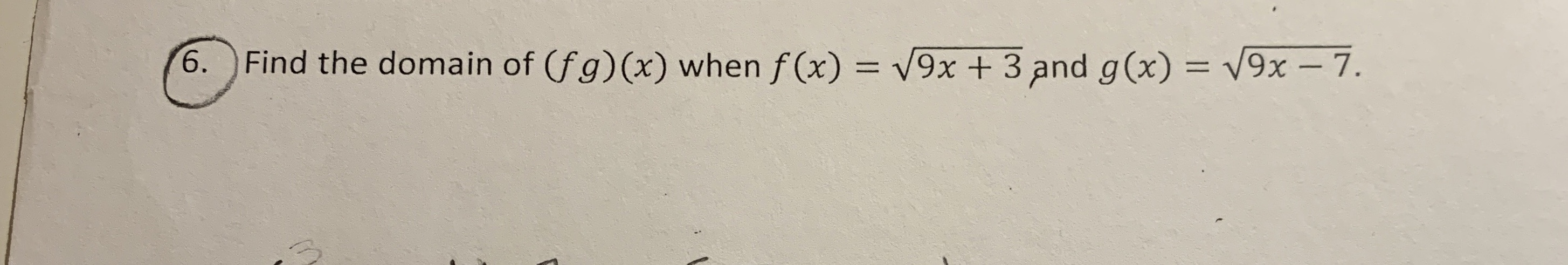# V9x +3 and g(x) = V9x- 7.6.Find the domain of (fg) (x) when f(x)

Question

How find the domain of composite function?help_outlineImage TranscriptioncloseV9x +3 and g(x) = V9x- 7. 6. Find the domain of (fg) (x) when f(x) fullscreen
check_circleExpert Solution
Step 1

Function are

Step 2

Let us assume the domain of f(x) is A and the domain of g(x) is B.

Thus,

Step 3

Now, finding the d...

### Want to see the full answer?

See Solution

#### Want to see this answer and more?

Solutions are written by subject experts who are available 24/7. Questions are typically answered within 1 hour*

See Solution
*Response times may vary by subject and question
Tagged in

### Numbers